# Kinetics: The Iodination of Propanone

5084 words (20 pages) Essay

26th Jan 2018 Chemistry Reference this

Disclaimer: This work has been submitted by a university student. This is not an example of the work produced by our Essay Writing Service. You can view samples of our professional work here.

Any opinions, findings, conclusions or recommendations expressed in this material are those of the authors and do not necessarily reflect the views of UKEssays.com.

This individual investigation will be exploring kinetics through experimentation the reaction: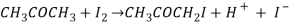This reaction is described as autocatalytic as it is produces the very species that increases the rate of reaction (hydrogen ions7).

## BackgroundReactants

Iodine is chemical that takes place in the reaction. Iodine is a halogen (group 7 on the periodic table) with a simple molecular covalent structure. Halogens are often diatomic as they have seven electrons on their outer shell (a full shell being eight) so to share electrons would allow them to be stable. Halogens are very electronegative elements meaning they are very likely to attract a bonding pair of electrons. As one if the less reactive halogens Iodine is a grey solid in standard conditions, this is due to it being a bigger so the outer electrons are further from the nucleus and thus harder for the attraction of an electron to form and Halide ion12.

The other halogens consist of Fluorine, Chlorine, Bromine and Astatine.

• Fluorine’s physical state is a pale yellow gas as it is the most reactive halogen. However it may not react with propanone in the same way as iodine as it does behave as a very good nucleophile. This is due to fluorine being less ready to share electrons with the electrophilic carbon in propanone.
• Chlorine’s physical state is a green gas and can be made into a virtually colourless solution but in this particular reaction will produce Chloroacetone (used as tear gas in the war) 10. This would put everyone is the laboratory at risk as tear gas can cause tearing, coughing and sneezing but inflaming mucous membranes in the eyes, nose and mouth. Changing the type of ketone in the reaction to butanone will prevent this haloketone from being produced, however the new ketone has a different chain length and therefore there is a need to test if there is a significant difference between the rates of the reaction the iodination of propanone and the iodination of butanone.
• Bromine’s physical state is a red-brown liquid and is yellow/orange in solution that reacts with propanone in a way that is analogous to iodine4. This halogen is more reactive than iodine as thus should result in a faster reaction rate12.
• Astatine cannot be used in this reaction as it is far too unreactive as well as radioactive.

Propanone (also known as acetone) is the most simple of ketones consisting of three carbons. Ketones are formed by refluxing secondary alcohols with acidified Potassium Dichromate Figure 4.

Products

Iodopropane is produced in the reaction

Spectator Ions

## Theory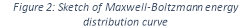In order for a reaction to take place particles need to collide, as occurs energy is shifted between them changing the amount of energy they have this is known as Collision Theory13. The distribution of this energy is displayed in a Maxwell-Boltzmann Curve Figure 2. The factors that have positive correlation with the rate constant3 and therefore effect the rate of a reaction do so because they affect the number of collisions (concentration) or the energy that each particle possesses (temperature) because this effects the number of particles that possess the activation energy (the minimum energy required for the reaction to take place)13. Catalysts also affect the rate of reaction by providing an alternative route for the reaction to occur; this route has a lower activation energy than the original reaction Figure 2 meaning more particles have enough energy to react thus increasing the reaction rate.

If a reaction has a great activation energy, the increase in temperature will have a large effect on the rate of reaction whereas if this activation energy was smaller, the change in temperature will be far less significant. This suggests that there is a relationship between reaction rate, temperature and activation energy. Due to this relationship the Arrhenius equation, using the ‘temperature dependence of the rate constant’ 13(k Figure 1), is applied to calculate activation energy of reaction via deriving a form of that fits y=mx+c (taking the natural logarithm).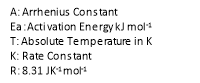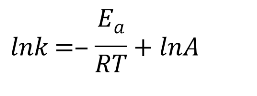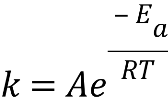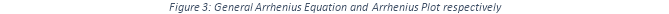Rate of reaction is defined as the speed at which reactants are formed into products or change in concentration over time taking into consideration the factors that affect it such as surface area, temperature and concentration13. There are many ways of measuring the concentration during a reaction but some are more efficient depending on the property that changes during the reaction. For example, the original reaction being investigated, the iodination of propanone, has an obvious colour change so a technique that detects absorbance (Colorimetry) would be a more efficient than measuring loss of mass when there is no gas released3.

With many techniques there arises the need to convert a particular property to concentration, in order to do so a calibration curve is needed. This curve is created by selecting known concentrations and measuring the level or amount of the property associated with the reaction and then drawing a property-concentration curve Figure x. Although it may be referred to as a curve more often than not it turns out to be rather linear as the concentrations used are rather low as the reactions would occur far too quickly if they were any higher, also high concentrations tend to have a higher hazard.

It is only through practical experiments that a rate equation giving the order of reactants can be written5.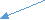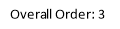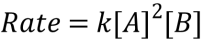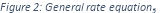• 0 order: Changing the concentration of this species has no effect on the rate of reaction.
• 1st order: The rate of reaction is directly proportional to the rate of reaction.
• 2nd order: As the concentration doubles the rate of reaction quadruples (exponential relationship).

The overall order of a reaction is calculated by adding the orders of each species Figure 1.

The order of a reaction with respect to A shows the number of molecules of A which are involved in the rate determining step. As a result of this a mechanism can be suggested if the rate equation is known as if the reactant is not in the rate equation it cannot feature in the rate determining step. The rate determining step is the slowest step in the reaction and thus it is responsible for the rate of the overall reaction. This step can be first or last (Bottleneck effect) and it would make no difference on the overall reaction rate.

## Aims

1. Explore the effects of different catalysts on the rate of the reaction and thus the activation energy.

After finding the rate equation for the original equation, the same experiment will be carried out with catalysts sulphuric, nitric and hydrochloric acid then with no catalyst (see reactions below). The activation energy can then be calculated via finding the different rates of reaction and thus the different values of the rate constant. This is all assuming the spectator ions have no effect on the reaction.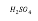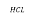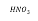1. Explore the effects of changing the type of halogen on the rate of the reaction.

The chosen halogens are Bromine and Chlorine. The following are the reactions that are going to take place as a result (respectively).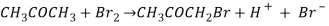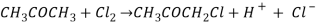1. Use rate equation to propose a rate determining step and explore a suggested mechanism.

If time permits exploring a suggested mechanism may consist of testing the presence of a product of one of the steps in the mechanism.

## Techniques

Colorimetry

• This technique will be used to calculate the order of the reaction between iodine and propanone.

This technique will also be used to measure the rates of the reactions between:

• Iodine and propanone
• Iodine and butanone
• Bromine and propanone
• Hypothesis: Although the ketones propanone and butanone have different formulas, the difference between the rates of reactions will be negligible and therefore the results for the reaction between chlorine with butanone and chlorine with propanone are more or less similar.
 Equipment List Chemical List 9 Boiling Tubes 10ml Pipette Spark Data logger Pipette Filler Colorimeter 20ml of 0.02 mol dm-3 Iodine 10ml of 0.02 mol dm-3 Bromine 20ml of 0.02 mol dm-3 Propanone 10ml of 0.02 mol dm-3 Butanone 30ml 0.02 mol dm-3 HCL Distilled water

Calibration Curve

1. Set up the colorimeter according to the instructions.
2. Place a cuvette full of distilled water inside the colorimeter and press the zero the button.
3. Select a suitable filter (gives your greatest concentration an absorption of close to one).
4. Make up 0.04, 0.016, 0.004, 0.0016, 0.0004, and 0.00016 mol dm-3 of iodine solution in test tubes by adding distilled water.
5. Label each test tube.
6. Place 6 ml 0.04 mol dm-3 of iodine solution in a cuvette and record the absorbance
7. Do the same for each of the other concentrations.
8. Draw a calibration curve by placing known concentrations of iodine solution in the colorimeter and recording the absorption.
9. Only repeat the concentrations that don’t seem to fit in the curve.

Method: To achieve Aim 1

1. Attach data logger to collect data automatically.
2. Place a cuvette full of distilled water inside the colorimeter and press the zero the button.
3. Select a suitable filter (gives your greatest concentration an absorption of close to one).
4. Mix sample A, sample B and 2 ml of 0.02 mol dm-3 HCL in a cuvette (add sample A last).
5. Immediately place cuvette inside the colorimeter and start recording absorbance at time intervals of 30 seconds for 6 minutes using the data logger.
6. Repeat Steps 2 to 5 two more times and calculate an average.
7. Use calibration curve to convert absorbance to concentration then draw a concentration-time graph.
 Sample A Sample B Experiment 1 2ml of 0.02 mol dm-3 Iodine 2ml of 0.02 mol dm-3 Propanone Experiment 2 2ml of 0.02 mol dm-3 Iodine 2ml of 0.02 mol dm-3 Butanone Experiment 3 2ml of 0.02 mol dm-3 Bromine 2ml of 0.02 mol dm-3 Propanone

Method: To achieve Aim 3 (Determine a rate equation)

1. Attach data logger to collect data automatically.
2. Place a cuvette full of distilled water inside the colorimeter and press the zero the button.
3. Select a suitable filter (gives your greatest concentration an absorption of close to one).
4. Add sample A, sample B and sample C in a cuvette.
5. Immediately after adding Xml of 0.02 mol dm-3 Iodine sol, place the cuvette inside the colorimeter.
6. Start recording absorbance at time intervals of 30 seconds for 6 minutes using the data logger.
7. Repeat Steps 2 to 6 two more times and calculate an average.
 Sample A Sample B Sample C X Experiment 1 0.9ml of 0.02 mol dm-3 Propanone 0.9ml of 0.02 mol dm-3 HCL 3.3ml distilled water 0.9 Experiment 2 1.8ml of 0.02 mol dm-3 Propanone 0.9ml of 0.02 mol dm-3 HCL 2.4ml distilled water 0.9 Experiment 3 0.9ml of 0.02 mol dm-3 Propanone 1.8ml of 0.02 mol dm-3 HCL 2.4ml distilled water 0.9 Experiment 4 0.9ml of 0.02 mol dm-3 Propanone 0.9ml of 0.02 mol dm-3 HCL 2.4ml distilled water 1.8
1. Use calibration curve to convert absorbance to concentration then draw a concentration-time graph.

Conductivity Meter11

• This technique will be used to measure and compare the reaction rates of the iodination of propanone with a different catalysts.
• Hypothesis: The catalyst with the most hydrogen ions available will have the fastest reaction of rate and thus the lowest activation energy. This is assuming the spectator ions have no effect on the conductivity meter.
 Equipment List Chemical List 100ml Beaker Conductivity Meter 10ml Pipette Pipette filler 2 Water baths Kettle/Bunsen Burner Test tubes 220cm3 of 0.02 mol dm-3 Propanone 120cm3 of 0.02 mol dm-3 HCL 60cm3 of 0.02 mol dm-3 H2SO4 220cm3 of 0.02 mol dm-3 HNO3 220cm3 of 1 mol dm-3 Iodine Distilled water

Method: To achieve Aim 3 (Effect of catalysts on activation energy)

1. Make two water baths (one large one small) at X degrees Celsius using a kettle and tap water.
2. Label 2 test tubes A and B then fill with 9ml of 0.02 mol dm-3 Propanone and 9ml of 0.02 mol dm-3 Iodine respectively.
 X Experiment 1 10 Experiment 2 20 Experiment 3 30 Experiment 4 40 Experiment 5 50 Experiment 6 60
1. Label 3 more test tubes X,Y and Z then fill with 3ml of 0.02 mol dm-3 H2SO4 , 3ml of 0.02 mol dm-3 HCL and 3ml of 0.02 mol dm-3 HNO3 respectively.
2. Place all of the test tubes in the small water bath for 2 minutes.
3. Place a beaker in the large water bath.
4. Pour 3 ml from test tubes A and X into the beaker.
5. Place conductivity probe in the beaker and adjust settings to consider the temperature of the water bath.
6. Add 3ml from test tube B into the beaker.
7. Start conductivity probe and record the conductivity every 30 seconds for 6 minutes.
8. Repeat steps 6 to 9 but using the contents of test tube Y.
9. Repeat steps 6 to 9 but using the contents of test tube Z.
10. Repeat steps 1 to 11 two more times and calculate an average.

Titration9, 12 14

• This technique will be used to measure the decrease in concentration of chlorine water throughout the reaction between chlorine solution and butanone in order to find the initial rate of reaction. As chlorine solution is virtually colourless it would be more effective to conduct an analysis that doesn’t rely on a colour change.
• A Redox titration will be used to measure the decrease in iodine concentration to ensure that the technique used to collect data doesn’t have a significant effect on the results. Sodium Hydrogen Carbonate will be used to quench the reaction as it removes the hydrogen ions from the reaction.
• Silver Nitrate Titration:
• The chloride ions produced by the reaction will react with the silver nitrate to form a white precipitate (silver chloride).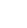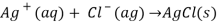• The end-point of the titration is a red precipitate caused by silver chloride reacting with the Sodium Chromate to form Silver Chromate(VI).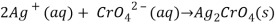• Redox Titration:
• The iodide ions produced by the reaction will oxidise the Sodium Thiosulphate, so the thiosulphate ions become tetrathionate ions in a redox reaction.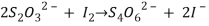• The solution will gradually start to go from brown to almost pale yellow. When this happens the starch indicator is added and reacts with triiodide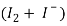forming a very dark blue-black complex. The end point is when this solution becomes colourless because it means that there is no longer any iodine left.
• Hypothesis: As Chlorine is more reactive than Iodine, the rate of the reaction should be faster. This is assuming that the technique has no significant effect on the results gained in this experiment.
 Equipment List Chemical ListDistilled Water 2 Conical Flasks Test tubes A White Tile Stopwatch Beaker White paper 50 ml of 1 mol dm-3 Sodium Hydrogen Carbonate Sodium Chromate 50 ml Silver Nitrate 100ml of 0.02 mol dm-3 Chlorine Butanone

Calibration Curve for Chlorine

1. Set up apparatus as seen in picture above.
2. Mark white sheet of paper with a large black cross.
3. Make up 10ml of 0.04, 0.016, 0.004, 0.0016, 0.0004, and 0.00016 mol dm-3 Chlorine solution in test tubes by adding distilled water.
4. Label each test tube.
5. For each test tube: Pour the contents of the test tube into a conical flask along with 1g Sodium Chromate dissolved in 20 ml distilled water.
6. Place conical flask on white sheet under burette.
7. Fill a burette with a recorded amount of 0.1 mol dm-3 Silver Nitrate solution.
8. Add drops of Silver Nitrate solution into the solution, swirling after every few drops, there will be a white precipitate formed.
9. Add more drops until the cross is no longer visible due to a brick red precipitate forming.
10. Record the new amount of Silver Nitrate in the burette.
11. Repeat steps 3 to 10 two more times and calculate an average titre (difference of 0.1).

Method: To achieve Aim 1 (Reaction rate of Chlorine and Butanone)

 X Experiment 1 1 Experiment 2 1.5 Experiment 3 2 Experiment 4 2.5 Experiment 5 3 Experiment 6 3.5 Experiment 7 4
1. Set up apparatus as seen in picture above.
2. Mark white sheet of paper with a large black cross.
3. Mix 3ml of 0.02 mol dm-3 Butanone, 3ml of 0.02 mol dm-3 HCL and 3ml of 0.02 mol dm-3 Chlorine in a conical flask.
4. After X minutes add 3ml of 1 mol dm-3 Sodium Hydrogen Carbonate to quench the reaction.
5. Add 1g Sodium Chromate dissolved in 20 ml distilled water to the conical flask.
6. Place conical flask on a white sheet under burette.
7. Fill a burette with a recorded amount of 0.1 mol dm-3 Silver Nitrate solution.
8. Add drops of Silver Nitrate solution into the solution, swirling after every few drops, there will be a white precipitate formed.
9. Add more drops until the cross is no longer visible due to a brick red precipitate forming.
10. Repeat steps 3 to 9 two more times and calculate an average titre (difference of 0.1).
11. Use calibration curve to obtain a concentration-time graph.

Calibration Curve for Iodine

1. Set up apparatus as seen in picture above.
2. Make up 10 ml of 0.04, 0.016, 0.004, 0.0016, 0.0004, and 0.00016 mol dm-3 iodine solution in test tubes by adding distilled water.
3. Label each test tube.
4. For each test tube: Pour the contents of the test tube into a conical flask.
6. Fill a burette with Sodium Thiosulphate, recording the amount.
7. Add drops of Sodium Thiosulphate into the solution, swirling after every few drops until there is a permanent colour change from a Black-Blue to colourless.
8. Record the new amount of Sodium Thiosulphate in the pipette.
9. Repeat steps 1 to 7 two more times and calculate an average titre (difference of 0.1).

Method: To achieve Aim 1 (Reaction rate of Iodine and Propanone)

1. Set up apparatus as seen in picture above.
2. Mix 3ml of 0.02 mol dm-3 Propanone, 3ml of 0.02 mol dm-3 HCL and 3ml of 0.02 mol dm-3 Iodine in a conical flask.
 X Experiment 1 1 Experiment 2 1.5 Experiment 3 2 Experiment 4 2.5 Experiment 5 3 Experiment 6 3.5 Experiment 7 4
1. After X minutes add 3ml of 1 mol dm-3 Sodium Hydrogen Carbonate to quench the reaction.
2. Add 3ml of Starch Indicator to the solution.
3. Fill a burette with Sodium Thiosulphate, recording the amount.
4. Add drops of Sodium Thiosulphate into the solution, swirling after every few drops until there is a permanent colour change from Blue-Black to colourless.
5. Record the new amount of Sodium Thiosulphate in the pipette.
6. Repeat steps 1 to 7 two more times and calculate an average titre (difference of 0.1).
7. Use calibration curve to obtain a concentration-time graph.

## Risk Assessment

 Chemical/ Procedure Hazard Risk Action if Occurred Precautions Risk Likelihood (out of 10) Colorimetry Titration (Precipitation) Use of a Conductivity Meter Propanone Butanone Iodine Chlorine Bromine Hydrochloric Acid Sulphuric Acid Nitric Acid Sodium Hydrogen Carbonate Iodopropanone Chlorobutanone Bromopropanone Iodine/Bromide /Chloride ions Sodium Nitrate Sodium Chromate Starch Indicator Sodium Thiosulphate

1 | Page

This individual investigation will be exploring kinetics through experimentation the reaction:This reaction is described as autocatalytic as it is produces the very species that increases the rate of reaction (hydrogen ions7).

## BackgroundReactants

Iodine is chemical that takes place in the reaction. Iodine is a halogen (group 7 on the periodic table) with a simple molecular covalent structure. Halogens are often diatomic as they have seven electrons on their outer shell (a full shell being eight) so to share electrons would allow them to be stable. Halogens are very electronegative elements meaning they are very likely to attract a bonding pair of electrons. As one if the less reactive halogens Iodine is a grey solid in standard conditions, this is due to it being a bigger so the outer electrons are further from the nucleus and thus harder for the attraction of an electron to form and Halide ion12.

The other halogens consist of Fluorine, Chlorine, Bromine and Astatine.

• Fluorine’s physical state is a pale yellow gas as it is the most reactive halogen. However it may not react with propanone in the same way as iodine as it does behave as a very good nucleophile. This is due to fluorine being less ready to share electrons with the electrophilic carbon in propanone.
• Chlorine’s physical state is a green gas and can be made into a virtually colourless solution but in this particular reaction will produce Chloroacetone (used as tear gas in the war) 10. This would put everyone is the laboratory at risk as tear gas can cause tearing, coughing and sneezing but inflaming mucous membranes in the eyes, nose and mouth. Changing the type of ketone in the reaction to butanone will prevent this haloketone from being produced, however the new ketone has a different chain length and therefore there is a need to test if there is a significant difference between the rates of the reaction the iodination of propanone and the iodination of butanone.
• Bromine’s physical state is a red-brown liquid and is yellow/orange in solution that reacts with propanone in a way that is analogous to iodine4. This halogen is more reactive than iodine as thus should result in a faster reaction rate12.
• Astatine cannot be used in this reaction as it is far too unreactive as well as radioactive.

Propanone (also known as acetone) is the most simple of ketones consisting of three carbons. Ketones are formed by refluxing secondary alcohols with acidified Potassium Dichromate Figure 4.

Products

Iodopropane is produced in the reaction

Spectator Ions

## TheoryIn order for a reaction to take place particles need to collide, as occurs energy is shifted between them changing the amount of energy they have this is known as Collision Theory13. The distribution of this energy is displayed in a Maxwell-Boltzmann Curve Figure 2. The factors that have positive correlation with the rate constant3 and therefore effect the rate of a reaction do so because they affect the number of collisions (concentration) or the energy that each particle possesses (temperature) because this effects the number of particles that possess the activation energy (the minimum energy required for the reaction to take place)13. Catalysts also affect the rate of reaction by providing an alternative route for the reaction to occur; this route has a lower activation energy than the original reaction Figure 2 meaning more particles have enough energy to react thus increasing the reaction rate.

If a reaction has a great activation energy, the increase in temperature will have a large effect on the rate of reaction whereas if this activation energy was smaller, the change in temperature will be far less significant. This suggests that there is a relationship between reaction rate, temperature and activation energy. Due to this relationship the Arrhenius equation, using the ‘temperature dependence of the rate constant’ 13(k Figure 1), is applied to calculate activation energy of reaction via deriving a form of that fits y=mx+c (taking the natural logarithm).Rate of reaction is defined as the speed at which reactants are formed into products or change in concentration over time taking into consideration the factors that affect it such as surface area, temperature and concentration13. There are many ways of measuring the concentration during a reaction but some are more efficient depending on the property that changes during the reaction. For example, the original reaction being investigated, the iodination of propanone, has an obvious colour change so a technique that detects absorbance (Colorimetry) would be a more efficient than measuring loss of mass when there is no gas released3.

With many techniques there arises the need to convert a particular property to concentration, in order to do so a calibration curve is needed. This curve is created by selecting known concentrations and measuring the level or amount of the property associated with the reaction and then drawing a property-concentration curve Figure x. Although it may be referred to as a curve more often than not it turns out to be rather linear as the concentrations used are rather low as the reactions would occur far too quickly if they were any higher, also high concentrations tend to have a higher hazard.

It is only through practical experiments that a rate equation giving the order of reactants can be written5.• 0 order: Changing the concentration of this species has no effect on the rate of reaction.
• 1st order: The rate of reaction is directly proportional to the rate of reaction.
• 2nd order: As the concentration doubles the rate of reaction quadruples (exponential relationship).

The overall order of a reaction is calculated by adding the orders of each species Figure 1.

The order of a reaction with respect to A shows the number of molecules of A which are involved in the rate determining step. As a result of this a mechanism can be suggested if the rate equation is known as if the reactant is not in the rate equation it cannot feature in the rate determining step. The rate determining step is the slowest step in the reaction and thus it is responsible for the rate of the overall reaction. This step can be first or last (Bottleneck effect) and it would make no difference on the overall reaction rate.

## Aims

1. Explore the effects of different catalysts on the rate of the reaction and thus the activation energy.

After finding the rate equation for the original equation, the same experiment will be carried out with catalysts sulphuric, nitric and hydrochloric acid then with no catalyst (see reactions below). The activation energy can then be calculated via finding the different rates of reaction and thus the different values of the rate constant. This is all assuming the spectator ions have no effect on the reaction.1. Explore the effects of changing the type of halogen on the rate of the reaction.

The chosen halogens are Bromine and Chlorine. The following are the reactions that are going to take place as a result (respectively).1. Use rate equation to propose a rate determining step and explore a suggested mechanism.

If time permits exploring a suggested mechanism may consist of testing the presence of a product of one of the steps in the mechanism.

## Techniques

Colorimetry

• This technique will be used to calculate the order of the reaction between iodine and propanone.

This technique will also be used to measure the rates of the reactions between:

• Iodine and propanone
• Iodine and butanone
• Bromine and propanone
• Hypothesis: Although the ketones propanone and butanone have different formulas, the difference between the rates of reactions will be negligible and therefore the results for the reaction between chlorine with butanone and chlorine with propanone are more or less similar.
 Equipment List Chemical List 9 Boiling Tubes 10ml Pipette Spark Data logger Pipette Filler Colorimeter 20ml of 0.02 mol dm-3 Iodine 10ml of 0.02 mol dm-3 Bromine 20ml of 0.02 mol dm-3 Propanone 10ml of 0.02 mol dm-3 Butanone 30ml 0.02 mol dm-3 HCL Distilled water

Calibration Curve

1. Set up the colorimeter according to the instructions.
2. Place a cuvette full of distilled water inside the colorimeter and press the zero the button.
3. Select a suitable filter (gives your greatest concentration an absorption of close to one).
4. Make up 0.04, 0.016, 0.004, 0.0016, 0.0004, and 0.00016 mol dm-3 of iodine solution in test tubes by adding distilled water.
5. Label each test tube.
6. Place 6 ml 0.04 mol dm-3 of iodine solution in a cuvette and record the absorbance
7. Do the same for each of the other concentrations.
8. Draw a calibration curve by placing known concentrations of iodine solution in the colorimeter and recording the absorption.
9. Only repeat the concentrations that don’t seem to fit in the curve.

Method: To achieve Aim 1

1. Attach data logger to collect data automatically.
2. Place a cuvette full of distilled water inside the colorimeter and press the zero the button.
3. Select a suitable filter (gives your greatest concentration an absorption of close to one).
4. Mix sample A, sample B and 2 ml of 0.02 mol dm-3 HCL in a cuvette (add sample A last).
5. Immediately place cuvette inside the colorimeter and start recording absorbance at time intervals of 30 seconds for 6 minutes using the data logger.
6. Repeat Steps 2 to 5 two more times and calculate an average.
7. Use calibration curve to convert absorbance to concentration then draw a concentration-time graph.
 Sample A Sample B Experiment 1 2ml of 0.02 mol dm-3 Iodine 2ml of 0.02 mol dm-3 Propanone Experiment 2 2ml of 0.02 mol dm-3 Iodine 2ml of 0.02 mol dm-3 Butanone Experiment 3 2ml of 0.02 mol dm-3 Bromine 2ml of 0.02 mol dm-3 Propanone

Method: To achieve Aim 3 (Determine a rate equation)

1. Attach data logger to collect data automatically.
2. Place a cuvette full of distilled water inside the colorimeter and press the zero the button.
3. Select a suitable filter (gives your greatest concentration an absorption of close to one).
4. Add sample A, sample B and sample C in a cuvette.
5. Immediately after adding Xml of 0.02 mol dm-3 Iodine sol, place the cuvette inside the colorimeter.
6. Start recording absorbance at time intervals of 30 seconds for 6 minutes using the data logger.
7. Repeat Steps 2 to 6 two more times and calculate an average.
 Sample A Sample B Sample C X Experiment 1 0.9ml of 0.02 mol dm-3 Propanone 0.9ml of 0.02 mol dm-3 HCL 3.3ml distilled water 0.9 Experiment 2 1.8ml of 0.02 mol dm-3 Propanone 0.9ml of 0.02 mol dm-3 HCL 2.4ml distilled water 0.9 Experiment 3 0.9ml of 0.02 mol dm-3 Propanone 1.8ml of 0.02 mol dm-3 HCL 2.4ml distilled water 0.9 Experiment 4 0.9ml of 0.02 mol dm-3 Propanone 0.9ml of 0.02 mol dm-3 HCL 2.4ml distilled water 1.8
1. Use calibration curve to convert absorbance to concentration then draw a concentration-time graph.

Conductivity Meter11

• This technique will be used to measure and compare the reaction rates of the iodination of propanone with a different catalysts.
• Hypothesis: The catalyst with the most hydrogen ions available will have the fastest reaction of rate and thus the lowest activation energy. This is assuming the spectator ions have no effect on the conductivity meter.
 Equipment List Chemical List 100ml Beaker Conductivity Meter 10ml Pipette Pipette filler 2 Water baths Kettle/Bunsen Burner Test tubes 220cm3 of 0.02 mol dm-3 Propanone 120cm3 of 0.02 mol dm-3 HCL 60cm3 of 0.02 mol dm-3 H2SO4 220cm3 of 0.02 mol dm-3 HNO3 220cm3 of 1 mol dm-3 Iodine Distilled water

Method: To achieve Aim 3 (Effect of catalysts on activation energy)

1. Make two water baths (one large one small) at X degrees Celsius using a kettle and tap water.
2. Label 2 test tubes A and B then fill with 9ml of 0.02 mol dm-3 Propanone and 9ml of 0.02 mol dm-3 Iodine respectively.
 X Experiment 1 10 Experiment 2 20 Experiment 3 30 Experiment 4 40 Experiment 5 50 Experiment 6 60
1. Label 3 more test tubes X,Y and Z then fill with 3ml of 0.02 mol dm-3 H2SO4 , 3ml of 0.02 mol dm-3 HCL and 3ml of 0.02 mol dm-3 HNO3 respectively.
2. Place all of the test tubes in the small water bath for 2 minutes.
3. Place a beaker in the large water bath.
4. Pour 3 ml from test tubes A and X into the beaker.
5. Place conductivity probe in the beaker and adjust settings to consider the temperature of the water bath.
6. Add 3ml from test tube B into the beaker.
7. Start conductivity probe and record the conductivity every 30 seconds for 6 minutes.
8. Repeat steps 6 to 9 but using the contents of test tube Y.
9. Repeat steps 6 to 9 but using the contents of test tube Z.
10. Repeat steps 1 to 11 two more times and calculate an average.

Titration9, 12 14

• This technique will be used to measure the decrease in concentration of chlorine water throughout the reaction between chlorine solution and butanone in order to find the initial rate of reaction. As chlorine solution is virtually colourless it would be more effective to conduct an analysis that doesn’t rely on a colour change.
• A Redox titration will be used to measure the decrease in iodine concentration to ensure that the technique used to collect data doesn’t have a significant effect on the results. Sodium Hydrogen Carbonate will be used to quench the reaction as it removes the hydrogen ions from the reaction.
• Silver Nitrate Titration:
• The chloride ions produced by the reaction will react with the silver nitrate to form a white precipitate (silver chloride).• The end-point of the titration is a red precipitate caused by silver chloride reacting with the Sodium Chromate to form Silver Chromate(VI).• Redox Titration:
• The iodide ions produced by the reaction will oxidise the Sodium Thiosulphate, so the thiosulphate ions become tetrathionate ions in a redox reaction.• The solution will gradually start to go from brown to almost pale yellow. When this happens the starch indicator is added and reacts with triiodideforming a very dark blue-black complex. The end point is when this solution becomes colourless because it means that there is no longer any iodine left.
• Hypothesis: As Chlorine is more reactive than Iodine, the rate of the reaction should be faster. This is assuming that the technique has no significant effect on the results gained in this experiment.
 Equipment List Chemical ListDistilled Water 2 Conical Flasks Test tubes A White Tile Stopwatch Beaker White paper 50 ml of 1 mol dm-3 Sodium Hydrogen Carbonate Sodium Chromate 50 ml Silver Nitrate 100ml of 0.02 mol dm-3 Chlorine Butanone

Calibration Curve for Chlorine

1. Set up apparatus as seen in picture above.
2. Mark white sheet of paper with a large black cross.
3. Make up 10ml of 0.04, 0.016, 0.004, 0.0016, 0.0004, and 0.00016 mol dm-3 Chlorine solution in test tubes by adding distilled water.
4. Label each test tube.
5. For each test tube: Pour the contents of the test tube into a conical flask along with 1g Sodium Chromate dissolved in 20 ml distilled water.
6. Place conical flask on white sheet under burette.
7. Fill a burette with a recorded amount of 0.1 mol dm-3 Silver Nitrate solution.
8. Add drops of Silver Nitrate solution into the solution, swirling after every few drops, there will be a white precipitate formed.
9. Add more drops until the cross is no longer visible due to a brick red precipitate forming.
10. Record the new amount of Silver Nitrate in the burette.
11. Repeat steps 3 to 10 two more times and calculate an average titre (difference of 0.1).

Method: To achieve Aim 1 (Reaction rate of Chlorine and Butanone)

 X Experiment 1 1 Experiment 2 1.5 Experiment 3 2 Experiment 4 2.5 Experiment 5 3 Experiment 6 3.5 Experiment 7 4
1. Set up apparatus as seen in picture above.
2. Mark white sheet of paper with a large black cross.
3. Mix 3ml of 0.02 mol dm-3 Butanone, 3ml of 0.02 mol dm-3 HCL and 3ml of 0.02 mol dm-3 Chlorine in a conical flask.
4. After X minutes add 3ml of 1 mol dm-3 Sodium Hydrogen Carbonate to quench the reaction.
5. Add 1g Sodium Chromate dissolved in 20 ml distilled water to the conical flask.
6. Place conical flask on a white sheet under burette.
7. Fill a burette with a recorded amount of 0.1 mol dm-3 Silver Nitrate solution.
8. Add drops of Silver Nitrate solution into the solution, swirling after every few drops, there will be a white precipitate formed.
9. Add more drops until the cross is no longer visible due to a brick red precipitate forming.
10. Repeat steps 3 to 9 two more times and calculate an average titre (difference of 0.1).
11. Use calibration curve to obtain a concentration-time graph.

Calibration Curve for Iodine

1. Set up apparatus as seen in picture above.
2. Make up 10 ml of 0.04, 0.016, 0.004, 0.0016, 0.0004, and 0.00016 mol dm-3 iodine solution in test tubes by adding distilled water.
3. Label each test tube.
4. For each test tube: Pour the contents of the test tube into a conical flask.
6. Fill a burette with Sodium Thiosulphate, recording the amount.
7. Add drops of Sodium Thiosulphate into the solution, swirling after every few drops until there is a permanent colour change from a Black-Blue to colourless.
8. Record the new amount of Sodium Thiosulphate in the pipette.
9. Repeat steps 1 to 7 two more times and calculate an average titre (difference of 0.1).

Method: To achieve Aim 1 (Reaction rate of Iodine and Propanone)

1. Set up apparatus as seen in picture above.
2. Mix 3ml of 0.02 mol dm-3 Propanone, 3ml of 0.02 mol dm-3 HCL and 3ml of 0.02 mol dm-3 Iodine in a conical flask.
 X Experiment 1 1 Experiment 2 1.5 Experiment 3 2 Experiment 4 2.5 Experiment 5 3 Experiment 6 3.5 Experiment 7 4
1. After X minutes add 3ml of 1 mol dm-3 Sodium Hydrogen Carbonate to quench the reaction.
2. Add 3ml of Starch Indicator to the solution.
3. Fill a burette with Sodium Thiosulphate, recording the amount.
4. Add drops of Sodium Thiosulphate into the solution, swirling after every few drops until there is a permanent colour change from Blue-Black to colourless.
5. Record the new amount of Sodium Thiosulphate in the pipette.
6. Repeat steps 1 to 7 two more times and calculate an average titre (difference of 0.1).
7. Use calibration curve to obtain a concentration-time graph.

## Risk Assessment

 Chemical/ Procedure Hazard Risk Action if Occurred Precautions Risk Likelihood (out of 10) Colorimetry Titration (Precipitation) Use of a Conductivity Meter Propanone Butanone Iodine Chlorine Bromine Hydrochloric Acid Sulphuric Acid Nitric Acid Sodium Hydrogen Carbonate Iodopropanone Chlorobutanone Bromopropanone Iodine/Bromide /Chloride ions Sodium Nitrate Sodium Chromate Starch Indicator Sodium Thiosulphate

1 | Page

## Related Services

View all

### DMCA / Removal Request

If you are the original writer of this essay and no longer wish to have your work published on the UKDiss.com website then please:

Related Services

Related Lectures